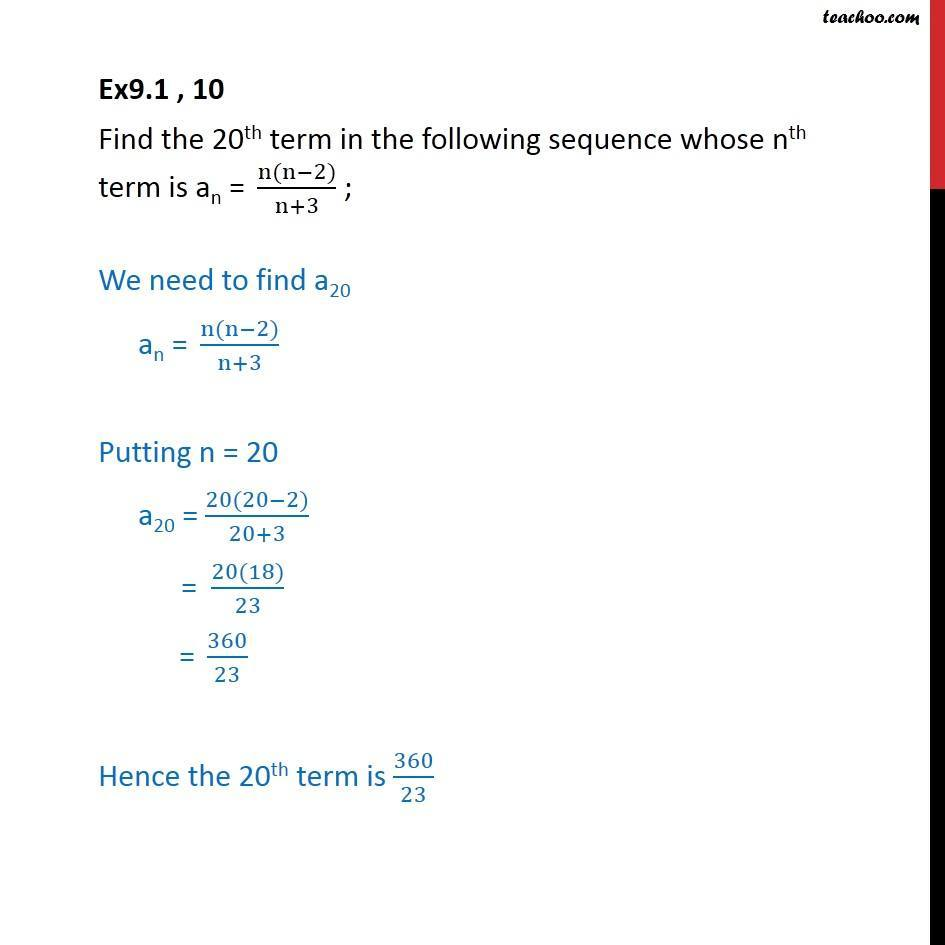Finding Sequences

Chapter 9 Class 11 Sequences and Series
Concept wiseIntroducing your new favourite teacher - Teachoo Black, at only ₹83 per month

### Transcript

Ex9.1 , 10 Find the 20th term in the following sequence whose nth term is an =" " (n(n−2))/(n+3) ; We need to find a20 an =" " (n(n−2))/(n+3) Putting n = 20 a20 = (20(20−2))/(20+3) = (20(18))/23 = 360/23 Hence the 20th term is 360/23